# Contraction of a representation

The contraction of a representationof a grouponto a subgroup(of an algebraonto a subalgebra) is the representationof the group (algebra)defined by the formula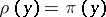for all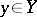. A contraction of a representationis also called a restriction (or reduction) of the representationonto an invariant subspace or onto a subgroup or subalgebra. Ifis a continuous representation, then a contraction ofis also continuous.
More usually one speaks of restriction of a representation and reduction of a representation. More precisely, if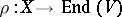is a representation of a group, algebraandis a subgroup, subalgebrathencan be restricted to. Secondly, ifis an invariant subspace of, theninduces a representation of(and) inyielding a subrepresentation of the given representation; this is sometimes called reduction of a representation to an invariant subspace. The phrase contraction of a representation refers more often to the following situation. Consider a representation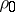over a ringof an algebra(or group) in an-module,. Suppose that there is a representationofover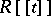,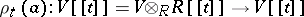such that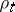(i.e.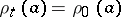for all). Heredenotes the module of power series inwith coefficients in. Then the representationis said to be a contraction ofandis called a deformation of. Intuitivelyis an (infinitesimal) family of representations parametrized by. More generally one also considers the situation where the algebrais also deformed at the same time, so that one has an algebraoversuch thatand a representationofover. Cf. also Deformation.